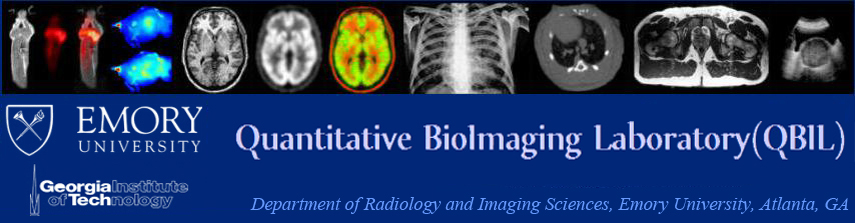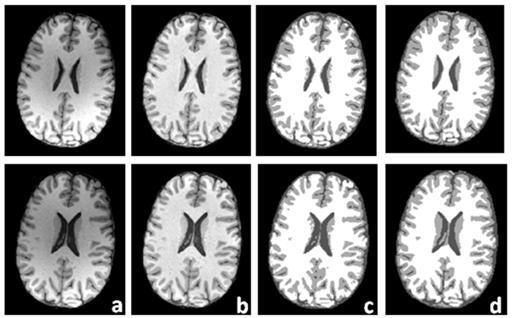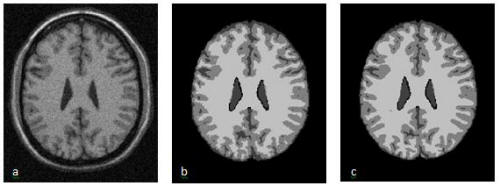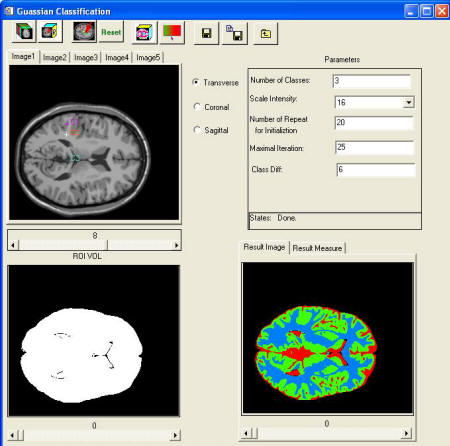Quantitative Image Analysis
Image Registration
Image Classification
Image Segmentation
Attenuation Correction
Partial Volume Correction
Motion Correction

Image classification is a process which partitions an image into a set of distinct classes with uniform or homogeneous attributes such as textures or intensity. Classification of images can be challenging because they are affected by multiple factors such as noise, poor contrast, intensity inhomogeneity, and partial volume effects. Classification methods can be categorized as supervised and unsupervised methods. Supervised classification methods depend on the examples of information classes in the images and derive a prior model or parameters from the training sets. Unsupervised methods examine the images without specific training data and divide the image pixels into groups presented in the pixel values according to the classification criteria.

Multiscale Image Classification

We developed a multiscale fuzzy c-means classification method for MR images. We used an anisotropic filter to effectively attenuate the noise within the images while still preserving the edges between different tissue types. A scale space was generated using anisotropic filtering, and the general structure information was kept in the images at a coarser scale. The classification was advanced along the scale space to include local information in the fine-level images. The result from a coarser scale provides the initial parameter for the classification in the next scale. Meanwhile, the pixels with a high probability of belonging to one class in the coarse scale will belong to the same class in the next level. Therefore, these pixels in the coarser images are considered as pixels with a known class and are used as the training data to constrain the classification in the next scale. In this way, we obtain accurate classifications step-by-step and avoid being trapped into local minima. Furthermore, we also include a regulation term which constrains the pixel so that it can be influenced by its immediate neighborhoods.Figure 8. Classification of brain MR image volumes. Results of two slices are showed in two rows: (a) the original MR images; (b) MR images after correction of MR intensity inhomogeneity; (c) is the classification where three gray levels represent CSF, gray matter and white matter; and (d) manual segmentations for validation of the classification method.Figure 9. The multi-scale classification software.

Gaussian Mixture Model-based Classification

We implemented an unsupervised classification method based on a finite Gaussian mixture model. The method assumes a Gaussian distribution for the intensity of each class. A single pixel is assigned to these classes with a probability based on image intensity. The problem is to determine the classification that optimally fits the image histogram, and it is solved using the expectation-maximization (EM) method. The final outcome is the probability of a pixel belonging to a class and the Gaussian description of each class intensity probability distribution. In order to take into account the strong spatial correlation between pixels, the Markov random field (MRF) is introduced into the classification. The Markov random field constrains the classification of a pixel depending on the major class of its neighboring pixels. This prior probability information is combined into the Bayesian framework as the Gibbs distribution for the EM algorithm.Figure 10. Classification results of brain MR images. (a) The original MR image, (b) the classified result, and (c) the ground truth for comparison.Figure 11. The Gaussian classification software.